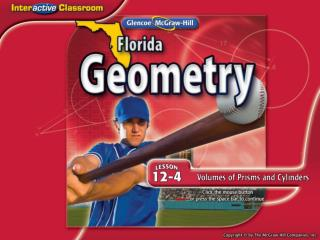DownloadDownload PresentationSplash Screen

# Splash Screen

Download Presentation## Splash Screen

- - - - - - - - - - - - - - - - - - - - - - - - - - - E N D - - - - - - - - - - - - - - - - - - - - - - - - - - -
##### Presentation Transcript

1. Splash Screen

2. Five-Minute Check (over Lesson 12–3) NGSSS Then/Now Key Concept : Volume of a Prism Example 1: Volume of a Prism Key Concept: Volume of a Cylinder Example 2: Volume of a Cylinder Key Concept: Cavalieri’s Principle Example 3: Volume of an Oblique Solid Example 4: Standardized Test Example Lesson Menu

3. A B C D Find the surface area of the regular pyramid. A. 600 in2 B. 623.2 in2 C. 635.3 in2 D. 647.4 in2 5-Minute Check 1

4. A B C D Find the surface area of the cone. A. 628.3 ft2 B. 642.3 ft2 C. 677.8 ft2 D. 753.9 ft2 5-Minute Check 2

5. A B C D Find the surface area of the regular pyramid. A. 420 ft2 B. 480 ft2 C. 679.8 ft2 D. 710 ft2 5-Minute Check 3

6. A B C D Find the surface area of the cone. A. 214.2 m2 B. 221.0 m2 C. 233.4 m2 D. 247.5 m2 5-Minute Check 4

7. A B C D Find the surface area of a square pyramid with base length of 10 feet and slant height of 8 feet. A. 80 ft2 B. 200 ft2 C. 240 ft2 D. 260 ft2 5-Minute Check 5

8. A B C D Find the lateral area of a cone with a diameter length of 10 centimeters and a slant height of 13 centimeters. Round your answer to the nearest tenth. A. 204.1 cm2 B. 282.7 cm2 C. 408.4 cm2 D. 722.6 cm2 5-Minute Check 6

9. MA.912.G.7.5Explain and use formulas for lateral area, surface area, and volume of solids. MA.912.G.7.7 Determine how changes in dimensions affect the surface area and volume of common geometric solids. NGSSS

10. You found surface areas of prisms and cylinders. (Lesson 12–2) • Find volumes of prisms. • Find volumes of cylinders. Then/Now

11. Concept

12. VBh Volume of a prism 1500 Simplify. Volume of a Prism Find the volume of the prism. Answer: The volume of the prism is 1500 cubic centimeters. Example 1

13. A B C D Find the volume of the prism. A. 6480 in3 B. 8100 in3 C. 3240 in3 D. 4050 in3 Example 1

14. Concept

15. = (1.8)2(1.8) r = 1.8 and h = 1.8 Volume of a Cylinder Find the volume of the cylinder to the nearest tenth. Volume of a cylinder ≈18.3 Use a calculator. Answer: The volume is approximately 18.3 cm3. Example 2

16. A B C D Find the volume of the cylinder to the nearest tenth. A. 62.8 cm3 B. 628.3 cm3 C. 125.7 cm3 D. 1005.3 cm3 Example 2

17. Concept

18. r 15, h 25 Use a calculator. Volume of an Oblique Solid Find the volume of the oblique cylinder to the nearest tenth. To find the volume, use the formula for a right cylinder. Volume of a cylinder Answer: The volume is approximately 17,671.5 cubic feet. Example 3

19. A B C D Find the volume of the oblique cylinder to the nearest tenth. A. 1520.5 cm3 B. 16,725.8 cm3 C. 5324 cm3 D. 8362.9 cm3 Example 3

20. Prisms A and B have the same width and height, but different lengths. If the volume of Prism B is 128 cubic inches greater than the volume of Prism A,what is the length of each prism? Prism A Prism B A 12 B 8 C 4 D 3.5 Example 4

21. Read the Test Item You know the volume of each solid and that the difference between their volumes is 128 cubic inches. Solve the Test Item Volume of Prism B – Volume of Prism A = 128 Write an equation. 4x● 9 – 4x● 5 = 128 Use V = Bh. 16x = 128 Simplify. x = 8 Divide each side by 16. Answer: The length of each prism is 8 inches. The correct answer is B. Example 4

22. A B C D Prisms A and B have the same width and height, but different lengths. If the volume of Prism B is 192 cubic inches greater than the volume of Prism A,what is the length of each prism? A. 4 in. B. 6 in. C. 8 in. D. 10.5 in. Prism A Prism B Example 4

23. End of the Lesson